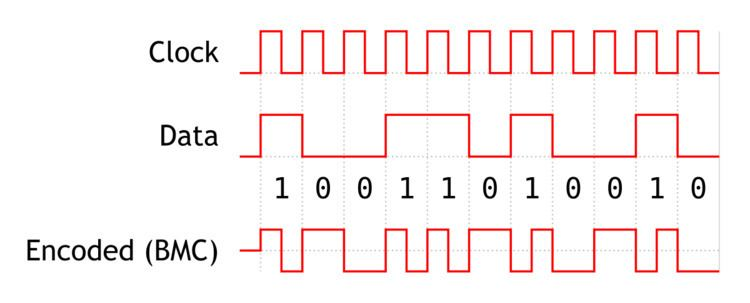# 4B3T

Updated on
Covid-194B3T, which stands for 4 (four) Binary 3 (three) Ternary, is a line encoding scheme used for ISDN PRI interface. 4B3T represents four binary bits using three pulses.

## Description

It uses three states:

• + (positive pulse),
• 0 (no pulse), and
• − (negative pulse).
• This means we have 24 = 16 input combinations to represent, using 33 = 27 output combinations. 000 is not used to avoid long periods without a transition. 4B3T uses a paired disparity code to achieve an overall zero DC bias: six triplets are used which have no DC component (0+−, 0−+, +0−, −0+, +−0, −+0), and the remaining 20 are grouped into 10 pairs with differing disparity (e.g. ++− and −−+). When transmitting, the DC bias is tracked and a combination chosen that has a DC component of the opposite sign to the running total.

This mapping from 4 bits to three ternary states is given in a table known as Modified Monitoring State 43 (MMS43). A competing encoding technique, used for the ISDN basic rate interface where 4B3T is not used, is 2B1Q.

The sync sequence used is the 11-symbol Barker code, +++−−−+−−+− or its reverse, −+−−+−−−+++.

## Encoding table

Each 4-bit input group is encoded as a 3-symbol group (transmitted left to right) from the following table. Encoding requires keeping track of the accumulated DC offset, the number of + pulses minus the number of − pulses in all preceding groups. The starting value is arbitrary; here we use the values 1 through 4, although −1.5, −0.5, +0.5 and +1.5 is another possibility.

This code forces a transition after at most five consecutive identical bits, or four consecutive zero bits.

## Decoding table

Decoding is simpler, as the decoder does not need to keep track of the encoder state, although doing so allows greater error detection. The 000 triplet is not a legal encoded sequence, but is typically decoded as binary 0000.

## References

Similar Topics
Freaky Friday (1976 film)
Bob Bainborough
Felino Palafox
Topics